Methods of expressing Concentration

Methods of expressing the concentration of Solution:-

The concentration of a solution means the amount of solute present in the given quantity of solution or solvent. the concentration of a solution may be expressed in the following ways

(note that symbol ‘A’ is used for Solute and ‘B’ is used for Solvent and ‘S’ for Solution). Generally liquid is always taken as solvent. if water is one of the components, it is taken as solvent.

1. Mass percentage and volume percentage:-

Mass percentage (%w/w) means the mass of solute in grams dissolved per 100 g of the solution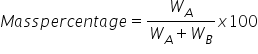Volume percentage (%w/w) means the volume of solute in ml dissolved per 100 ml of the solution.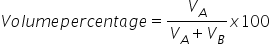However if nothing is expressed, it is taken as % by mass. If mass is to be converted into volume and density is not given, it is taken as 1 g/cm3

2.Normality:- Normality of a solution is defined as the number of gram equivalents of the solute dissolved per liter of the solution. It is represented by N:

Mathematically: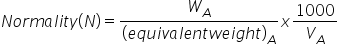The equivalent weight of the solute is calculated as: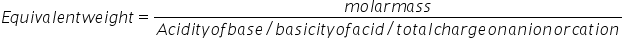Basicity of acid is the no. of H+ furnished by it e.g. HCl, HNO3, CH3COOH has basicity 1 and H2SO4 has 2. Similarly acidity of base is the number of OH- given by it e.g. NaOH, KOH, have acidity 1, Ca(OH)2 has 2 and Al (OH)3 has acidity 3.

A solution having normally equal to one is called a normal solution, a semi-normal solution has normality ½ and a decinormal solution has normalty 1/10.

The unit of normalty is gm eq L-1

3.Molarity:- Molarity of a solution is defined as the number of moles of solute dissolved per liter (or dm3) of the solution. It is represented by ‘M’. the units of molarity are moles L-1.

Mathematically: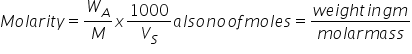Relationship between molarity and normality:

Normalty x eq. mass (solute) = Molarity x molar mass (solute)

Or           Normalty = acidity or Basicity x Molarity

4.Molality (m):  Molality of a solution may be defined as the no. of moles of solute dissolved per kilogram (1000 gm) of the solvent. it is represented by ‘m’. Mathematically: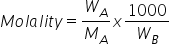5)Mole Fraction:- Mole fraction of a component in solution is the ratio of no. of moles of that component to the total on. Of moles of all the components in solution. It is denoted by ‘x’ let two components A and B with molar mass MA and MB having weights WA and WB from the solution.

No. of moles of A = nA

No. of moles of B = nB

Total no. of moles in solution = nA + nB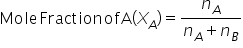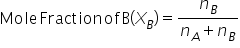The sum of mole fractions of all the components in the solution is always unify i.e. 1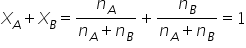Mole fraction is dimension less quantity and has no units.

6)Parts per million (ppm):- it is the number of parts by mass of solute dissolved per million parts by mass of solution.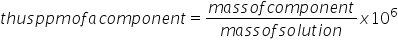This term is used for very dilute solutions.

Related Keywords